Engineering ToolBox - Resources, Tools and Basic Information for Engineering and Design of Technical Applications!

# Beams - Supported at Both Ends - Continuous and Point Loads

## Supporting loads, stress and deflections.

The stress in a bending beam can be expressed as

σ = y M / I                                     (1)

where

σ = stress (Pa (N/m2), N/mm2, psi)

y = distance to point from neutral axis (m, mm, in)

M = bending moment (Nm, lb in)

I = moment of Inertia (m4, mm4, in4)

The calculator below can be used to calculate maximum stress and deflection of beams with one single or uniform distributed loads.

### Beam Supported at Both Ends - Uniform Continuous Distributed Load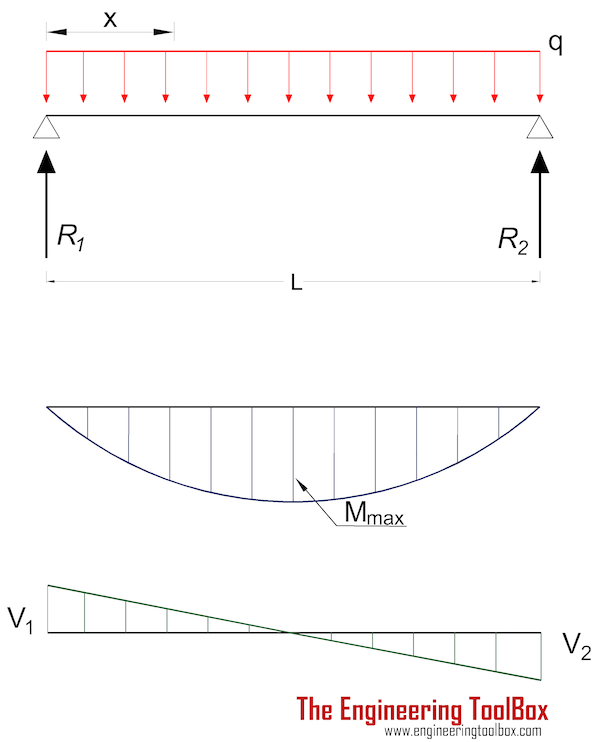The moment in a beam with uniform load supported at both ends in position x can be expressed as

Mx = q x (L - x) / 2                                          (2)

where

Mx = moment in position x (Nm, lb in)

x = distance from end (m, mm, in)

The maximum moment is at the center of the beam at distance L/2 and can be expressed as

Mmax = q L2 / 8                                          (2a)

where

Mmax = maximum moment (Nm, lb in)

q = uniform load per length unit of beam (N/m, N/mm, lb/in)

L = length of beam (m, mm, in)

#### Maximum Stress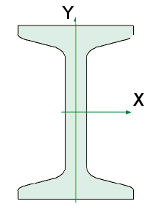Equation 1 and 2a can be combined to express maximum stress in a beam with uniform load supported at both ends at distance L/2 as

σmax = ymax q L2 / (8 I)                                     (2b)

where

σmax= maximum stress (Pa (N/m2), N/mm2, psi)

ymax = distance to extreme point from neutral axis (m, mm, in)

• 1 N/m2 = 1x10-6 N/mm2 = 1 Pa = 1.4504x10-4 psi
• 1 psi (lb/in2) = 144 psf (lbf/ft2) = 6,894.8 Pa (N/m2) = 6.895x10-3 N/mm2

Maximum deflection:

δmax = 5 q L4 / (384 E I)                                     (2c)

where

δmax = maximum deflection (m, mm, in)

E = Modulus of Elasticity (Pa (N/m2), N/mm2, psi)

Deflection in position x:

δx = q x (L3 - 2 L x2 + x3/ (24 E I)                                     (2d)

Note! - deflection is often the limiting factor in beam design. For some applications beams must be stronger than required by maximum loads, to avoid unacceptable deflections.

Forces acting on the ends:

R1 = R2

= q L / 2                                      (2e)

where

R = reaction force (N, lb)

#### Example - Beam with Uniform Load, Metric Units

UB 305 x 127 x 42 beam with length 5000 mm carries a uniform load of 6 N/mm. The moment of inertia for the beam is 8196 cm4 (81960000 mm4) and the modulus of elasticity for the steel used in the beam is 200 GPa (200000 N/mm2). The height of the beam is 300 mm (the distance of the extreme point to the neutral axis is 150 mm).

The maximum stress in the beam can be calculated

σmax = (150 mm) (6 N/mm) (5000 mm)2 / (8 (81960000 mm4))

= 34.3 N/mm2

= 34.3 106 N/m2 (Pa)

= 34.3 MPa

The maximum deflection in the beam can be calculated

δmax = 5 (6 N/mm) (5000 mm)4 / ((200000 N/mm2) (81960000 mm4) 384)

= 2.98 mm

#### Uniform Load Beam Calculator - Metric Units

L - Length of Beam (mm)

I - Moment of Inertia (mm4)

E - Modulus of Elasticity (N/mm2)

y - Distance of extreme point off neutral axis (mm)

• 1 mm4 = 10-4 cm4 = 10-12 m4
• 1 cm4 = 10-8 m = 104 mm
• 1 in4 = 4.16x105 mm4 = 41.6 cm4
• 1 N/mm2 = 106 N/m2 (Pa)

#### Uniform Load Beam Calculator - Imperial Units

L - Length of Beam (in)

I - Moment of Inertia (in4)

E - Modulus of Elasticity (psi)

y - Distance of extreme point off neutral axis(in)

#### Example - Beam with Uniform Load, Imperial Units

The maximum stress in a "W 12 x 35" Steel Wide Flange beam, 100 inches long, moment of inertia 285 in4, modulus of elasticity 29000000 psi, with uniform load 100 lb/in can be calculated as

σmax = ymax q L2 / (8 I)

= (6.25 in) (100 lb/in) (100 in)2 / (8 (285 in4))

= 2741 (lb/in2, psi)

The maximum deflection can be calculated as

δmax = 5 q L4 / (E I 384)

= 5 (100 lb/in) (100 in)4 / ((29000000 lb/in2) (285 in4) 384)

= 0.016 in

### Beam Supported at Both Ends - Load at Center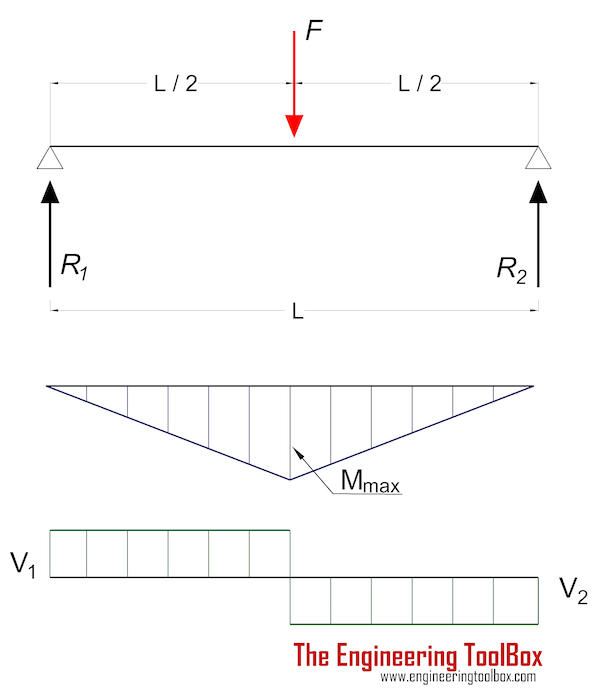Maximum moment in a beam with center load supported at both ends:

Mmax = F L / 4                                          (3a)

#### Maximum Stress

Maximum stress in a beam with single center load supported at both ends:

σmax = ymax F L / (4 I)                                    (3b)

where

Maximum deflection can be expressed as

δmax = F L3 / (48 E I)                                  (3c)

Forces acting on the ends:

R1 = R2

= F / 2                                      (3d)

#### Single Center Load Beam Calculator - Metric Units

L - Length of Beam (mm)

I - Moment of Inertia (mm4)

E - Modulus of Elasticity (N/mm2)

y - Distance of extreme point off neutral axis (mm)

#### Single Center Load Beam Calculator - Imperial Units

L - Length of Beam (in)

I - Moment of Inertia (in4)

E - Modulus of Elasticity (psi)

y - Distance of extreme point off neutral axis (in)

#### Example - Beam with a Single Center Load

The maximum stress in a "W 12 x 35" Steel Wide Flange beam, 100 inches long, moment of inertia 285 in4, modulus of elasticity 29000000 psi, with a center load 10000 lb can be calculated like

σmax = ymax F L / (4 I)

= (6.25 in) (10000 lb) (100 in) / (4 (285 in4))

= 5482 (lb/in2, psi)

The maximum deflection can be calculated as

δmax = F L3 / E I 48

= (10000 lb) (100 in)3 / ((29000000 lb/in2) (285 in4) 48)

= 0.025 in

### Some Typical Vertical Deflection Limits

• total deflection : span/250
• live load deflection : span/360
• cantilevers : span/180
• domestic timber floor joists : span/330 (max 14 mm)
• brittle elements : span/500
• crane girders : span/600

### Beam Supported at Both Ends - Eccentric Load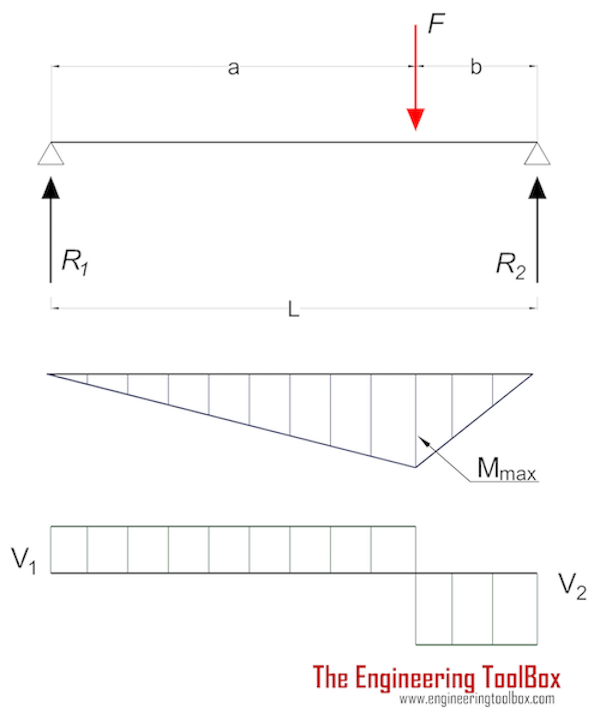Maximum moment in a beam with single eccentric load at point of load:

Mmax = F a b / L                                          (4a)

#### Maximum Stress

Maximum stress in a beam with single center load supported at both ends:

σmax = ymax F a b / (L I)                                    (4b)

Maximum deflection at point of load can be expressed as

δF = F a2 b2 / (3 E I L)                                  (4c)

Forces acting on the ends:

R1 = F b / L                                 (4d)

R2 = F a / L                                 (4e)

### Beam Supported at Both Ends - Two Eccentric Loads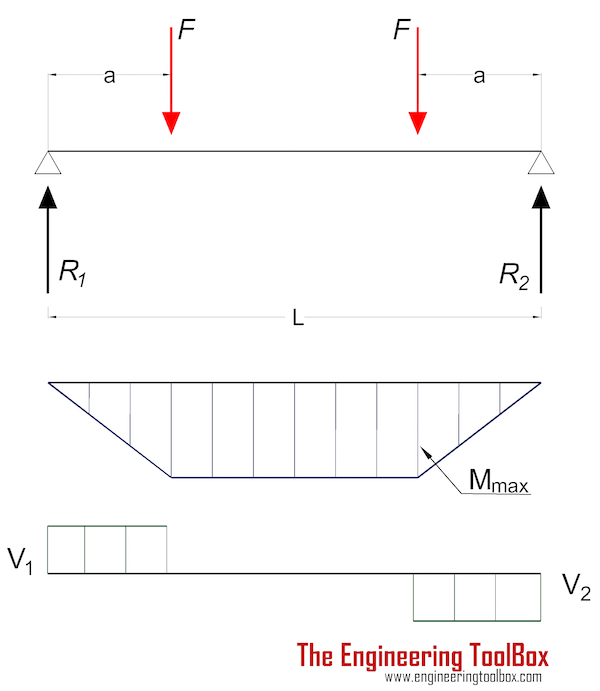Mmax = F a                                          (5a)

#### Maximum Stress

Maximum stress in a beam with two eccentric loads supported at both ends:

σmax = ymax F a / I                                    (5b)

Maximum deflection at point of load can be expressed as

δF = F a (3L2 - 4 a2) / (24 E I)                                  (5c)

Forces acting on the ends:

R1 = R2

= F                                  (5d)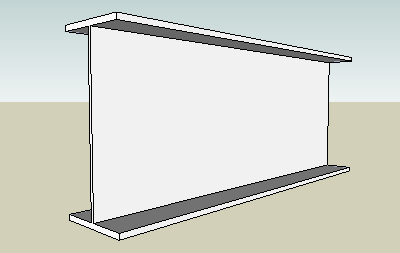Insert beams to your Sketchup model with the Engineering ToolBox Sketchup Extension

### Beam Supported at Both Ends - Three Point Loads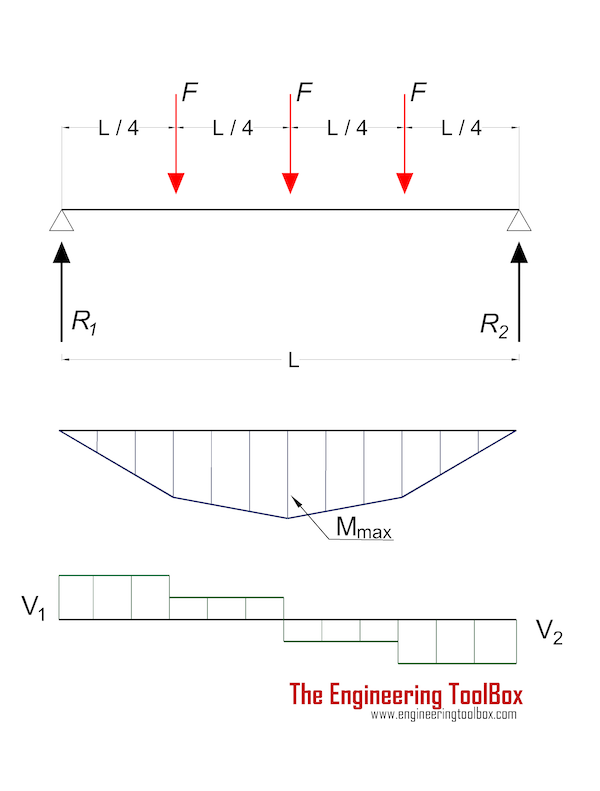Mmax = F L / 2                                          (6a)

#### Maximum Stress

Maximum stress in a beam with three point loads supported at both ends:

σmax = ymax F L / (2 I)                                    (6b)

Maximum deflection at the center of the beam can be expressed as

δF = F L3 / (20.22 E I)                               (6c)

Forces acting on the ends:

R1 = R2

= 1.5 F                                  (6d)

## Related Topics

• ### Beams and Columns

Deflection and stress, moment of inertia, section modulus and technical information of beams and columns.
• ### Mechanics

Forces, acceleration, displacement, vectors, motion, momentum, energy of objects and more.
• ### Statics

Loads - forces and torque, beams and columns.

## Related Documents

• ### Aluminum I-Beams

Dimensions and static properties of aluminum I-beams - Imperial units.
• ### American Standard Beams - S Beam

American Standard Beams ASTM A6 - Imperial units.
• ### American Standard Steel C Channels.

Dimensions and static parameters of American Standard Steel C Channels
• ### American Wide Flange Beams

American Wide Flange Beams ASTM A6 in metric units.
• ### Area Moment of Inertia - Typical Cross Sections I

Typical cross sections and their Area Moment of Inertia.
• ### Area Moment of Inertia - Typical Cross Sections II

Area Moment of Inertia, Moment of Inertia for an Area or Second Moment of Area for typical cross section profiles.
• ### Area Moment of Inertia Converter

Convert between Area Moment of Inertia units.
• ### Beam Loads - Support Force Calculator

Calculate beam load and supporting forces.

• ### Beams Natural Vibration Frequency

Estimate structures natural vibration frequency.
• ### British Universal Columns and Beams

Properties of British Universal Steel Columns and Beams.
• ### Cantilever Beams - Moments and Deflections

Maximum reaction forces, deflections and moments - single and uniform loads.
• ### Continuous Beams - Moment and Reaction Support Forces

Moments and reaction support forces with distributed or point loads.
• ### Drawbridge - Force and Moment vs. Elevation

Calculate the acting forces and moments when elevating drawbridges or beams.
• ### Floor Joist Capacities

Carrying capacities of domestic timber floor joists - Grade C - metric units.
• ### Floors - Live Loads

Floors and minimum uniformly distributed live loads.
• ### HE-A Steel Beams

Properties of HE-A profiled steel beams.
• ### HE-B Steel Beams

Properties of HE-B profiled steel beams.
• ### HE-M Steel Beams

Properties of HE-M profile steel beams.
• ### Normal Flange I-Beams

Properties of normal flange I profile steel beams.
• ### Section Modulus - Unit Converter

Convert between Elastic Section Modulus units.
• ### Square Hollow Structural Sections - HSS

Weight, cross sectional area, moments of inertia - Imperial units
• ### Steel Angles - Equal Legs

Dimensions and static parameters of steel angles with equal legs - imperial units.
• ### Steel Angles - Equal Legs

Dimensions and static parameters of steel angles with equal legs - metric units.
• ### Steel Angles - Unequal Legs

Dimensions and static parameters of steel angles with unequal legs - imperial units.
• ### Steel Angles - Unequal Legs

Dimensions and static parameters of steel angles with unequal legs - metric units.
• ### Stiffness

Stiffness is resistance to deflection.
• ### Stress

Stress is force applied on cross-sectional area.
• ### Three-Hinged Arches - Continuous and Point Loads

Support reactions and bending moments.
• ### Trusses

Common types of trusses.

• ### W-Beams - American Wide Flange Beams

Dimensions of American Wide Flange Beams ASTM A6 (or W-Beams) - Imperial units.
• ### Weight of Beams - Stress and Strain

Stress and deformation of vertical beams due to own weight.
• ### Wood Headers - Max. Supported Weight

Weight supported by a double or triple wood headers.

## Engineering ToolBox - SketchUp Extension - Online 3D modeling!

Add standard and customized parametric components - like flange beams, lumbers, piping, stairs and more - to your Sketchup model with the Engineering ToolBox - SketchUp Extension - enabled for use with older versions of the amazing SketchUp Make and the newer "up to date" SketchUp Pro . Add the Engineering ToolBox extension to your SketchUp Make/Pro from the Extension Warehouse !

We don't collect information from our users. More about

## Citation

• The Engineering ToolBox (2009). Beams - Supported at Both Ends - Continuous and Point Loads. [online] Available at: https://www.engineeringtoolbox.com/beam-stress-deflection-d_1312.html [Accessed Day Month Year].

Modify the access date according your visit.

9.19.12